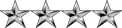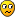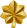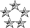## Need help To Binomial Theorem And Binomial Coefficients

Mathematics for Computing
Nipuna
ModeratorPosts: 2729
Joined: Mon Jan 04, 2010 8:02 pm
Location: Deraniyagala,SRI LANKA

### Need help To Binomial Theorem And Binomial Coefficients

What are Binomial Theorem And Binomial Coefficients?

No Much Exploitations in VLEHerath
MajorPosts: 417
Joined: Thu Aug 05, 2010 7:09 pm

### Re: Need help To Binomial Theorem And Binomial CoefficientsCapture.PNG (21.71 KiB) Viewed 3234 times
http://en.wikipedia.org/wiki/Binomial_theorem
NeoPosts: 2642
Joined: Wed Jul 15, 2009 2:07 am
Location: Colombo

### Re: Need help To Binomial Theorem And Binomial Coefficients

Binomial theorem describes the algebraic expansion of powers of a binomial. According to the theorem, it is possible to expand the power (x + y)n into a sum involving terms of the form axbyc, where the exponents b and c are non-negative integers with b + c = n, and the coefficient a of each term is a specific positive integer depending on n and b. When an exponent is zero, the corresponding power is usually omitted from the term.

I'll give you a quick guide.pt.png (4.12 KiB) Viewed 3229 times
Say you have to expand the binomial (x + y)3.

Step 1: Write x terms from power 3 to 0.
x3 + x2 + x1 + x0

Step 2: Add y terms from power 0 to 3 after the x term (In reverse order).
x3y0 + x2y1 + x1y2 + x0y3

Step 3: Remove power 0 terms (x0 = y0 = 1). Also write power 1 terms without power sysmbol (x1 = x, y1 = y)
x3 + x2y + xy2 + y3

Step 4: Add coefficients (සංගුනක) according to Pascal Triangle for Power 3 (4th (3+1) raw) since we are expanding (x + y)3.
1 3 3 1 are the values from Pascal Triangle for Power 3 (4th raw). These are called binomial coefficients.
1x3 + 3x2y + 3xy2 + 1y3

Finally we get:
(x + y)3 = x3 + 3x2y + 3xy2 + y3

Let's evaluate (a + b)5 quickly now.
Step 1and 2 together:
a5b0 + a4b1 + a3b2 + a2b3 + a1b4 + a0b5

Step 3:
a5 + a4b + a3b2 + a2b3 + ab4 + b5

Step 4:
Chose the binomial coefficients from Pascal triangle. We need the value for Power 5. So we look at the 6th raw.
1 5 10 10 5 1
1a5 + 5a4b + 10a3b2 + 10a2b3 + 5ab4 + 1b5

Finally we have,
(a + b)5 = a5 + 5a4b + 10a3b2 + 10a2b3 + 5ab4 + b5

I guess you are now clear on the process.